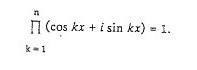# Solve equation with unknown x

## Homework Statement

I have to solve the following equation:## The Attempt at a Solution

I know that since the right side is 1 and on the left side i have i (imaginary number) it means that i could rewrite right side as cos0 + isin0 since it's the same, but what can i do with left side, it obviously can't stay like this because of the product, i have to get rid of it, i just don't have an idea.

Samy_A
Homework Helper
What do you get when you replace the factors in the product using Euler's formula?

What do you get when you replace the factors in the product using Euler's formula?

As far as i know, we have Пekx (П is from 1 to n) which is equal to:

ex*e2x*...*enx or

e(1+2+...+n)x. Now, i am not 100% sure, but i believe is should represent 1+2+...+n as n(n+1)/2 and go back to the trigonometric form of number, and then simply find x since i know exact value of angle for the right side. Am i correct?

Samy_A
Homework Helper
As far as i know, we have Пekx (П is from 1 to n) which is equal to:

ex*e2x*...*enx or

e(1+2+...+n)x. Now, i am not 100% sure, but i believe is should represent 1+2+...+n as n(n+1)/2 and go back to the trigonometric form of number, and then simply find x since i know exact value of angle for the right side. Am i correct?
You forgot the ##i## in your exponent, as Euler's formula is ##cos(y)+isin(y)=e^{iy}##.
You are correct about ##\sum_{k=1}^n k =n(n+1)/2##.
As the product is equal to ##1##, you can now indeed solve for x by applying Euler's formula again: the cosine of your exponent (not including the ##i##) must be ##1##.

Last edited:
RUber
Homework Helper
How many solutions do you need? Just one, all of them for a fixed n, or all of them for any n?
##e^{iy} = 1 ## is true for an infinite number of periodic terms.
For a large w, ##e^{iwy} ## will have a much shorter period. Your w might get quite large as n grows.